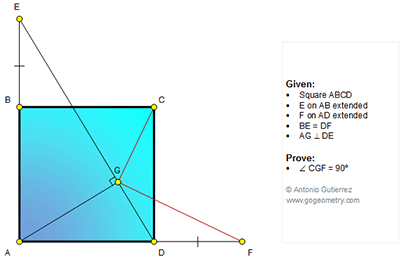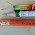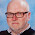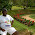## Thursday, July 16, 2015

### Geometry Problem 1138: Square, Perpendicular, Angle, 90 Degrees, Triangle, Congruence

Geometry Problem. Post your solution in the comments box below.
Level: Mathematics Education, High School, Honors Geometry, College.

Click the diagram below to enlarge it.#### 7 comments:

1.Join C to E & F and observe that triangles EBC & FDC are congruent. Hence, /_BEC=/_DFC -------(1).
From Tr. EAD, we can say that DG*DE= AD²=DC². In other words, DC is tangent to the circum-circle of Tr.GEC and, therefore, we can see that /_GEC=/_GCD (Alternate segment theorem). Moreover, /_BEG=/_GDC since BE//DC. Thus, /_BEC=/_BEG+/_GEC= /_GDC+/_GCD= /_EGC. But by (1) above, /_BEC=/_DFC. This means that /_EGC = /_DFC implying that quad.GCFD is concyclic. Then, /_CGF=/_CDF=90°. QED.

1.Got almost all your steps Ajit without realising that DC is tangential to GEC Tr. Hence could not complete

Well done

2.That, in fact, is what took me almost two hours to realize. Otherwise, I was planning to do this by analytical geometry in this fashion: Let A:(0,0), D:(a,0),F:(a+p,0), C:(a,a) and B:(0,a+p). ED can be written as: x/a+y/(a+p)=1 while AG is: y=ax/(a+p) which gives us
G as :[a(a+p)²/(a²+(a+p)²), a²(a+p)/(a²+(a+p)²)]. Now we determine slope of CG as [(a²+p²+ap) /a²] and that of GF as [-a²/(a²+p²+ap) ] , the product of which is -1. Hence etc.
But this solution misses all the niceties, the congruence of two triangles, the tangency and the consequent concyclicity etc of the solution by Euclidean elements.

3.Just leaving a breadcrumb for . The crucial tangency of DC and Tr. GEC comes from the power of a point theorem.

2.E, F are symmetrical about AC, so if H is intersection of EF with AC, EF_|_AC, and EG.ED=AE^2=EH.EF, so GDFH is cyclic, as well as DFCH, hence CH_|_GF.

3.Extend AG and BC ; intersect at P.
tr. APB = tr. DEA ( AE=BP => CP=DF
DFPC is parallellogram ( CP//DEF and CP=DF ) ; DFPC is rectangle thus concyclic
GDCP is concyclic
DPFC and GDCP concyclic => GDFCP concyclic => <CGF=<CDF=90

4.If EF and AC cut at X then
AXE, AXF and AEF are all 45-45-90 Tr.s

So AEXG is concyclic and
< XGC = < AEX = 45 = < EAX = EGX = < EFD

Hence XGDF is concyclic and so
< CGF = CXF = 90

Sumith Peiris
Moratuwa
Sri Lanka